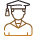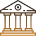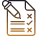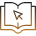# IOQJS 5 Year Analysis: Which Topics Get More Weightage And Why

###### BySafeer PP###### Synopsis

The goal of IOQJS, is to test the experimental skills, logical reasoning and problem-solving abilities of students  of Class 8, 9 or 10. Qualifying the IOQJS with top scores opens the way to the International Olympiad. Besides the recognition of their merit in Physics, Chemistry and Math, successful students can assess their chances in tougher entrance exams like JEE or NEET to secure a career in science or technology. Ace IOQJS by reading the 5-year analysis that tells you from which topics maximum questions were asked and the chapters with maximum weightage from Class 10 to 12.###### Synopsis

The goal of IOQJS, is to test the experimental skills, logical reasoning and problem-solving abilities of students  of Class 8, 9 or 10. Qualifying the IOQJS with top scores opens the way to the International Olympiad. Besides the recognition of their merit in Physics, Chemistry and Math, successful students can assess their chances in tougher entrance exams like JEE or NEET to secure a career in science or technology. Ace IOQJS by reading the 5-year analysis that tells you from which topics maximum questions were asked and the chapters with maximum weightage from Class 10 to 12.

The National Olympiad in Junior Science is conducted by the Indian Association of Physics Teachers (IAPT) and the Homi Bhabha Center for Science Education (HBCSE). The aim of the National Olympiad in Junior Science is to understand the experimental skill, logical reasoning and problem-solving skills of the students. The IOQJS exam is usually conducted in two stages – National Standard Examination in Junior Science (NSEJS) and Indian National Junior Science Olympiad (INJSO). Top 35 to 50 students of the Indian National Olympiads are selected for orientation and selection camp at HBCSE. At least 4 to 6 students from the selection camp are provided a pre-departure camp before the International Olympiad, the final stage. Gold medal and merit certificates are provided by IAPT for all the students who are selected for the orientation-cum-selection camp. Certificates are provided for top 10% students from each centre of INJSO and 1% of state and national toppers of IOQJS will get a certificate that can be downloaded from iapt.org.

IOQJS Exam 2021-2022 exam was held on 6th March 2022. The exam was conducted in two stages on the same day – the IOQJS Part 1 (NSEJS) and Part 2 (INJSO). The top 35 to 50 students of IOQJS will be selected for the third stage, that is, the Orientation-cum-Selection Camps (OCSCs).

From the official website students can download the previous year’s INJSO papers and model solutions. The syllabus of IOQJS is not exactly similar to the NCERT Science and Mathematics syllabus of Class 9 and 10. The syllabus also includes some advanced topics that are listed in this article. The basic understanding of Mathematics and Science up to Class 10 is also required for NSEJS and INJSO. The question paper till 2020-2019 had questions from Mathematics and Science and the paper conducted in March 2022 does not have questions from Mathematics. But there are aptitude type questions that require basic understanding of Mathematics up to the secondary level.

## 5-Year Analysis of IOQJS Part 1 (NSEJS)

The analysis is done for 2021, 2020, 2019, 2018 and 2016 NSEJS papers for both Mathematics and Science parts. The 2020 and 2021 NSEJS papers have two sections. The first section has multiple choice questions (MCQs) with a single answer and the second section has MCQs with more than 1 correct answer. In section 1 a wrong answer will attract deduction of 1/3rd mark but section 2 does not have negative marking. The other papers of NSEJS have only objective questions with single correct answers. The Science part is divided into three for the analysis, namely Physics, Chemistry and Biology. Each question of Part 1 carries 3 marks and Part 2 carries 6 marks. The weightage is calculated based on the marks distribution for each chapter.

### Physics

The chapters coming under Class 10 and 9 Physics are – Light Reflection and Refraction; The Human Eye and the Colourful World; Electricity; Magnetic Effect of Electric Current; Motion; Force and Laws of Motion; Work and Energy; Gravitation and Sound. Other than the syllabus of NCERT Class 9 and 10 students should focus on experimental skills related to the chapters. The weightage is calculated by accounting all the subjects. That is, Electricity has a weightage of 6.21% out of all the 4 subjects and corresponding chapters.

Table: NSEJS Physics 5- Year Paper Analysis: Chapter-wise

 Chapter No. of Questions Weightage % Basic Mathematics 1 0.31% Electricity 18 6.21% Force and Laws of Motion 5 1.55% Gravitation 11 3.42% Human Eye and Colourful World 1 0.31% Light Reflection and Refraction 15 4.66% Magnetic Effects of Electric Current 5 1.86% Motion 4 1.24% Sound 5 1.55% Thermal Properties of matter 5 1.55% Work and Energy 8 2.80%

Some important topics to be studied other than NCERT Class 9 and 10 chapters are listed below. The list is selected according to the previous 5-year papers. These topics listed are also useful for IOQP Part 2 (INJSO).

Simple Pendulum: Time Period And Energy of The Pendulum; Damping Oscillations and Spring Constant. In the analysis these topics are tagged with Work And Energy.

Thermal Properties of Matter: Relation Between Fahrenheit And Celsius; Heat Transfer; Black Body Radiation; Heat Capacity and Latent Heat. The basics of Thermal Properties are studied in Class 7, but NSEJS requires a bit more topics to be covered and these can be found in the books mentioned in the official website and also by referring to previous year’s questions of NSEJS.

Electrostatics: Concept of Charge, Coulomb's Law; Properties of Electric Field and Electrostatic Shielding. For analysis Electrostatics is tagged with Class 10 NCERT Science chapter on Electricity.

Dimensional Analysis: Dimensional Formula and Dimensional Analysis.

Properties of Fluids: Pascal's Law and Anomalous Behaviour of Water. In the analysis these topics are tagged with the chapter, Gravitation, of Class 9.

Relations between Planck's constant and energy are also to be noted --E=h?. Here E is the energy and ? is the frequency.

Important chapters to be focused are: Electricity; Light Reflection and Refraction; Work and Energy; and Gravitation. The chapter, Electricity, has the highest weightage from Physics part of Science syllabus for NSEJS. Some problems in Physics only require basic mathematical ideas to solve it.

### Chemistry

The chapters mentioned in the syllabus of the NSEJS belong to Class 9 and Class 10, however, after analysing the last 5 years' question papers it is found that many questions are based on the concepts of Class 11 and Class 12 Chemistry. It would be beneficial for students to cover at least the basic concepts provided in Class 11 and 12 NCERT Chemistry. Apart from this, analytical skills are highly required as some questions are purely based on common sense which can be solved without using any core concept of Chemistry. Hence, a mix of knowledge and logical mindset is required to ace the NSEJS examination.

NSEJS 5-Year Chemistry Paper Analysis: Chapter-wise

 Chapter Total Number of Questions Weightage% Acids, Bases And Salts 14 4.66% Atoms And Molecules 9 3.11% Carbon And Its Compounds 7 2.17% Chemical Reactions And Equations 19 6.21% Is Matter Around Us Pure 8 2.48% Matter In Our Surroundings 2 0.62% Metals And Non-Metals 8 2.80% Periodic Classification of Elements 11 3.73% Structure of The Atom 1 0.31%

As mentioned above, in previous years, there were questions which were not from NCERT Class 9 and 10 Chemistry chapters. Below mentioned are some of the topics which students can refer from Class 11 and 12 in order to improve their score in NSEJS examination.

Solutions: Basic concepts related to Molarity, Molality, Mole Fraction Etc. ; Vapour Pressure, Colligative Properties, Osmotic Pressure.

Chemical Bonding: Types of Bonding; Polarity of Bonds,; Hybridisation; Structures of Molecules; Bonding in Diatomic Molecules; Molecular Orbital Theory.

States of Matter: Intermolecular Forces; Gaseous Laws; Ideal Gas Equation,;Partial Pressure; Liquid State; Composition of Gas at normal temperature and pressure; Behaviour of Real Gases and Deviation From Ideal Gas Behaviour.

Some Basic Concepts of Chemistry: Atomic and Molecular Masses; Law of Chemical Combination; Concentration; Molar Weight and Equivalent Weight; Percentage Composition; Stoichiometric and Non- Stoichiometric Calculations.

Equilibrium: Types of Chemical Equilibrium; Equilibrium Constant; Factors Affecting Equilibrium; Importance of Equilibrium.

Hydrocarbons: Physical and Chemical Properties of Alkanes; Alkenes and Alkynes; Chemical Reaction of Hydrocarbons which includes Ozonolysis, and Hydroboration, among others.

Polymers: Name of Various Polymers; Classification of Polymers; Physical Properties of Various Polymeric Compounds; and Chemical Reactions Involving Polymers.

Students are recommended to go through last 5 years' question papers of NSEJS and try to attempt in exam-like conditions to acquaint themselves with the difficulty level of the examination. Proper analysis of difficulty level of questions is an important part of preparation. Students can score decent marks if all the topics mentioned above are prepared well, along with the syllabus of Class 9 and Class 10.

### Biology

According to the NSEJS 5-year papers analysis, the most important chapters as per weightage according to frequency of questions asked are given below. The Fundamental Unit of Life; Heredity and Evolution; and Life Processes are the three main chapters from which the highest numbers of questions were asked. The NCERT enables you to build your foundation conceptually, however, studying only the NCERT book will not be sufficient to qualify the exam. Careers360’s advice is that you study these related topics from higher classes to obtain a good score as this exam analyses your experimental skills, high order thinking and how you can link the topics from different chapters.

Table: NSEJS 5-year Biology Paper Analysis: Chapter-wise

 Chapter No. Of Questions Weightage % Control and Coordination 5 1.86% Diversity in Living Organisms 10 3.11% Heredity and Evolution 18 5.90% How Do Organisms Reproduce? 5 1.55% Improvement in Food Resources 2 0.62% Life Processes 16 5.28% Natural Resources 4 1.24% Our Environment 4 1.24% The Fundamental Unit of Life 10 3.42% Tissues 2 0.62% Why Do We Fall Ill? 7 2.48%

You might encounter some questions that do not match from Class 9 and 10 NCERT books. For example, some questions from Heredity and Evolution; Life Process; Cell Cycle and Division; Locomotion and Movement; Hormones, Biomolecules, Immune System; and Biotechnology were levelled up, and you need to study these topics from Class 12 NCERT or other related books. Apart from this, there were some out of syllabus questions that analyse your other skills. For example, there were questions (only 1 or 2) from behavioural science. Some questions were based on lab experiments too. So, do not avoid activities given in the books.

Experimental thinking while studying the concepts is a must for getting a good score.

### Mathematics

Class 9 and 10 Mathematics syllabuses of NSEJS include Real Numbers, Number System, Polynomials, Pair of Linear Equations in Two Variables, Quadratic Equations, Arithmetic Progressions, Lines and Angles, Triangles, Coordinate Geometry, Introduction to Trigonometry, Some Applications of Trigonometry, Circles, Areas Related to Circles, Quadrilaterals, Areas of Parallelograms and Triangles, Surface Areas and Volumes, Statistics, Probability. According to the analysis done by Careers360’s subject specialists, questions are being asked frequently from the concepts of chapters i.e. Real Number, Arithmetic Progressions, Probability, Polynomials, Quadratic Equations, Trigonometry. Trigonometry (Introduction To Trigonometry And Application of Trigonometry) both combined, account for the second important topic with a weightage of 2.48%. Maximum questions are being asked from the topic Real Number, which accounts for 3.42%. Chapter-wise weightage is given below.

NSEJS 5-Year Mathematics Paper Analysis: Chapter-wise

 Chapter No. of Questions Weightage % Areas of Parallelograms and Triangles 3 0.93% Areas Related to Circles 1 0.31% Arithmetic Progressions 7 2.17% Circles 4 1.24% Introduction to Trigonometry 4 1.55% Number Systems 2 0.62% Pair of Linear Equations In Two Variables 5 1.55% Polynomials 6 1.86% Probability 7 2.17% Quadratic Equations 4 1.55% Quadrilaterals 1 0.31% Real Numbers 11 3.42% Some Applications of Trigonometry 3 0.93% Surface Areas and Volumes 3 0.93% Triangles 5 1.55%

Some questions require a basic understanding of concepts taught in Class 11 and 12 to solve them. Therefore, students are advised to go through the basics concepts given in Class 11 and 12 NCERT books . Some topics from which questions have been asked are listed below:

Functions and Relations: Type of Functions; Greatest Integer Function; Mod Function

Linear Inequalities: Concepts to Solve Inequalities

Trigonometry: Solutions of Trigonometry Equations

Students are advised to go through the previous year’s question papers to get an idea of the difficulty level.

## 5-Year Analysis of IOQJS Part 2 (INJSO)

INJSO (Indian National Junior Science Olympiad) is the second stage of IOQJS exam. The analysis is done for 2021, 2020, 2019, 2018, and 2017 INJSO papers. The pattern of the paper has been changed twice in this period. The 2017 and 2018 papers were divided into two sections: section A for objective (MCQs) questions and section B for subjective questions. Section A had a negative marking of ¼ for each wrong answer. In 2019, the pattern was the same but negative marking was changed to 1/3 for each wrong answer. In 2020, the paper was divided into three sections: Section I for objective (MCQs), section II for multiple select questions (MSQs), and section III for subjective questions and negative marking was kept the same at1/3 for each wrong answer. In 2021, the paper was divided into two sections -: section I for objective questions (MCQs) and section II for subjective questions including true/or false statements. The negative marking was for objective questions in all these years but in 2021 it was introduced for subjective questions as well (for true/false statements only). Careers360’s prediction is that the upcoming INJSO paper might have 2 sections- objective and subjective or 3 sections – MCQ, MSQs, and subjective questions. So, prepare yourself by keeping that in your mind.

The INJSO paper includes three subjects: Physics, Chemistry, and Biology. The subject-wise analysis is given below:

### Biology

According to the INJSO-5-year papers analysis, the most important chapter as per weightage is Life Processes as this chapter covers most parts of Plant Physiology and Animal Physiology. However, questions were levelled up and you need to read the books from higher classes as well. The numbers of questions with weightage from the chapters are given below:

INJSO 5-year Biology Paper Analysis: Chapter-wise

 Chapter Number of Questions Weightage% Control and Coordination 4 1.56% Diversity In Living Organisms 5 1.1% Heredity and Evolution 9 5.32% How Do Organisms Reproduce? 2 1.25% Improvement in Food Resources 1 0.47% Life Processes 22 14.69% Our Environment 6 6.09% The Fundamental Unit of Life 7 2.34% Tissues 2 0.31% Why Do We Fall Ill? 3 1.09%

The Fundamental Unit of Life; Heredity and Evolution; and Our Environment are the other chapters from which the highest numbers of questions were asked. A number of questions were experiment-based, graph-based and figure-based to analyse your distinct skills. Suggestions remain the same as the previous one: study these related topics from higher classes to get a good score as this exam analyzes your in depth knowledge, experimental skills, high order thinking, and how you can link the topics from different chapters.

### Chemistry

The Table given below provides chapter-wise weightage of INJSO in the last 5 years’ papers. It is clear from the Table that barring chapters – Atoms and Molecules and Matter In Our Surroundings – all other chapters have to be covered comprehensively. Considering the level of competition, it is recommended to cover all the chapters as there might be an increase in the weightage from less asked chapters in the upcoming years.

INJSO 5-year Chemistry Paper Analysis: Chapter-wise

 Chapter Number of Questions Weightage% Acids Bases and Salts 12 8.52% Atoms and Molecules 1 0.16% Carbon and its Compounds 3 2.19% Chemical Reactions and Equations 12 7.19% Is Matter Around Us Pure 9 4.14% Matter In Our Surroundings 2 0.94% Metals and Non-Metals 7 2.34% Periodic Classification of Elements 5 3.75% Structure of The Atom 6 3.13%

From the above Table of chemistry chapters’ weightage, it is clear that the chapters – Acids,Bases and Salts And Chemical Reactions and Equations –have the maximum weightage. Hence, covering these topics is indispensable to maximise the score.

### Physics

INJSO 5-year Physics Paper Analysis: Chapter-wise

 Chapter Number of Questions Weightage% Electricity 16 6.88% Force and Laws of Motion 3 2.97% Gravitation 4 2.19% Light Reflection and Refraction 15 7.34% Magnetic Effects of Electric Current 2 0.63% Motion 10 5.00% Sound 5 4.53% Thermal Properties of Matter 6 3.44% Work and Energy 3 0.47%

The highest weightage is for the Class 10 chapter, Light Reflection and Refraction (7.34%). More questions are asked from the chapter Electricity that includes topics of Electrostatics also.For basic understanding of topics in Electrostatics students can refer to Class 12 NCERT Physics. From Electricity, 16 questions with a weightage of 6.88% were there. Topics like Motion and Sound are also important. Among all the three subjects, the highest weightage is for the chapter, Life Process. All the three parts of Class 10 Science (Physics, Chemistry and Biology) are given almost equal weightage for IOQJS exam.

• Chemistry
• Physical sciences
• Applied and interdisciplinary physics
• Physical chemistry
• Physics
• Physical quantities
• Molecule
• Gas
• Polymer
• Chemical equilibrium

## Careers360 helping shape your Career for a better tomorrow#### 250M+

Students#### 30,000+

Colleges#### 500+

ExamsE-Books